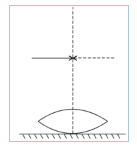# A symmetric biconvex lens of radius of curvature R and made of glass of refractive index 1·5, is placed on a layer of liquid placed on top of a plane mirror as shown in the figure. An optical needle with its tip on the principal axis of the lens is moved along the axis until its real, inverted image coincides with the needle itself. The distance of the needle from the lens is measured to be x. On removing the liquid layer and repeating the experiment, the distance is found to be y. Obtain the expression for the refractive index of the liquid in terms of x and y.Given,
Refractive index of lens
Distance of needle from the lens = focal length of systems = x = f
Distance after removing liquid layer = focal length of lens = y = f1
Let, focal length of liquid = f2
refractive index of liquid =
The equivalente focal length f is

Given that radius of Curvature of other surfaces of the lens  is -R
from lens maker formula

Since the liquid act as a plane mirror, therefore
Applying lens maker formula for liquid, we get

Therefore, the expression for the refractive index of the liquid is

### Preparation Products

##### Knockout NEET Sept 2020

An exhaustive E-learning program for the complete preparation of NEET..

₹ 15999/- ₹ 6999/-
##### Rank Booster NEET 2020

This course will help student to be better prepared and study in the right direction for NEET..

₹ 9999/- ₹ 4999/-
##### Knockout JEE Main Sept 2020

An exhaustive E-learning program for the complete preparation of JEE Main..

₹ 12999/- ₹ 6999/-
##### Test Series NEET Sept 2020

Take chapter-wise, subject-wise and Complete syllabus mock tests and get in depth analysis of your test..

₹ 4999/- ₹ 2999/-